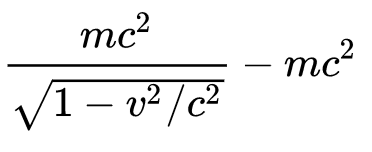# History of Idea of Energy

Back to Energy

#### From Afterthought to Alternative to Central Role

• Afterthought
• Newtonian Mechanics, Sir Isaac Newton, 1687
• Newton did not use the concept of energy in his Mechanics.
• But Leibniz, a contemporary of Newton, developed the idea of kinetic energy, which he called vis viva, defining it as mv2. He proved that vis visa was conserved in inelastic collisions.
• Alternative
• Lagrangian Mechanics, Joseph-Louis Lagrange, 1788
• Lagrange developed an abstract, alternative, top-down formulation of Newtonian Mechanics.  Its central concept was the Lagrangian: kinetic energy minus potential energy.
• Hamiltonian Mechanics, Sir William Rowan Hamilton, 1833
• Hamilton, like Lagrange, developed an abstract, alternative, top-down formulation of Newtonian Mechanics.  Its central concept was the Hamiltonian: kinetic energy plus potential energy, i.e. total energy.
• Central Role
• Quantum Mechanics, 1925
• Though very different from Newton Mechanics, Quantum Mechanics retained the concept of the Hamiltonian, kinetic energy plus potential energy, which in QM is governed by the Schrodinger equation iℏ ∂|Ψ⟩/∂t = H|Ψ⟩, where H is the Hamiltonian.

#### Timeline

###### 1687 Isaac Newton developed Newtonian Mechanics
• Central concept is Force, which equals Mass X Acceleration
• Newtonian Mechanics predicts the motion of falling bodies, the orbits of planets, the movement of pendulums, and the trajectory of cannon balls
• Newton did not use the concept of energy in his Mechanics.
###### 1689 Gottfried Wilhelm Leibniz introduced the idea of kinetic energy
• Leibniz and Newton independently invented calculus
• Leibniz introduced the idea of vis viva (living force), which he defined as mv2
• The formula is like that for kinetic energy, except for missing the ½.
• Vis viva is conserved in inelastic collisions.
###### 1689-1788 Ideas of Potential Energy and the Conservation of Energy gradually emerged
• The kinetic energy of a ball thrown straight up in the air disappears and then reappears on the way down.  Scientists thought this strange. Over the course of a century a theory gradually emerged:
• A second form of energy exists: potential energy (energy of location)
• Kinetic energy + potential energy is conserved.
• Scientists needed to develop a formula for potential energy.
• 18th century engineers, analyzing the comparative efficiency of windmills, waterwheels, and various kinds of steam engines, had developed the concept of work as force times distance, e.g. how high an engine could lift so many pounds of water
• Potential Energy = work done to move an object from a reference point to a certain location, e.g. gravitational potential energy = -GmM/r
###### 1788 Joseph-Louis Lagrange published Mécanique Analytique (“Analytic Mechanics”)
• “By 1761 Lagrange was recognized as one of the greatest living mathematicians.”
• Lagrangian Mechanics is an abstract, generalized, top-down formulation of Newtonian Mechanics
• A physical system is governed by one equation:
• ∂L/∂x = d/dt (∂L/∂v)
• where L is the Lagrangian, which equals kinetic energy minus potential energy.
###### 1833  William Rowan Hamilton published  “On a General Method in Dynamics”
• Hamiltonian Mechanics, like Lagrangian Mechanics, is an abstract, generalized, top-down formulation of Newtonian Mechanics
• A physical system is governed by two equations
• dpi/dt = -∂H/∂qi
• dqi/dt = ∂H/∂pi
• where H is the Hamiltonian, which equals kinetic energy plus potential energy.
• That is, H is the total energy of the system
###### 1850s Thermodynamics was developed
• A new form of energy is recognized
• Internal Energy is the energy of a system by virtue of the kinetic and potential energies of its atoms, molecules, electrons, and other particles.
• Heat is recognized as a form of energy transfer, like work.
###### 1865 James Clerk Maxwell developed the Theory of Electromagnetism
• New forms of energy are recognized
• Electric Potential Energy
• Energy stored in an electric field
• Magnetic Potential Energy
• Energy stored in a magnetic field
• Energy transported in an electromagnetic wave
###### 1905 Albert Einstein published papers on Special Relativity and E = mc2
• Einstein revises the formula for kinetic energy
•• That is, the kinetic energy of a particle equals its total energy minus a new form of energy: mass energy
• The mass energy E of a particle is mc2
• where m is the particle’s mass (at rest) and c is the speed of light.
• The kinetic energy of cars, trains, and planes is effectively ½ mv2
• The kinetic energy of a particle moving faster than 1/100 the speed of light exceeds ½ mv2
• A collision between an electron and its antiparticle (the positron) results in their simultaneous annihilation, their masses being converted into energy in the form of gamma rays per E = mc2
###### 1920s Quantum Mechanics was developed
• Quantum Mechanics is radically different from Classical Physics
• QM does not track particles through space and time
• QM generates probabilistic predictions
• QM uses discrete (quantized) quantities
• A physical system evolves according to the Schrodinger Equation
• ℏ ∂|Ψ⟩/∂t = H|Ψ⟩
• where H is the Hamiltonian, which equals kinetic energy plus potential energy